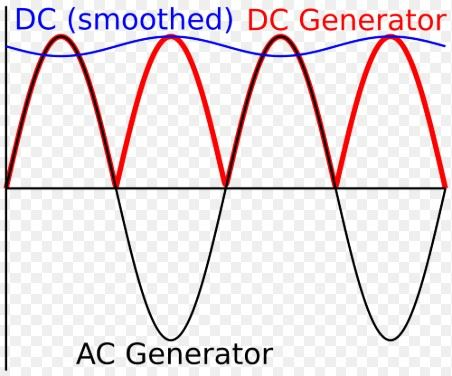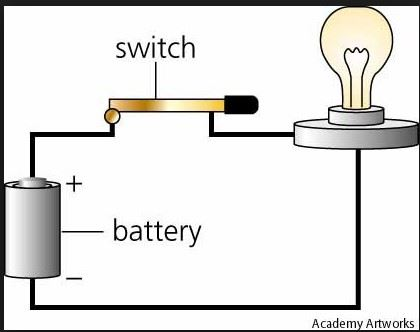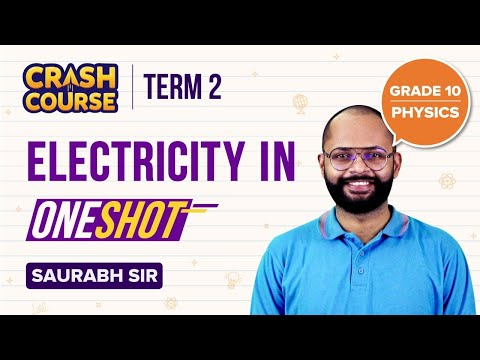Checkout JEE MAINS 2022 Question Paper Analysis : Checkout JEE MAINS 2022 Question Paper Analysis :

# Current Electricity PDF

## What is the Electric Current?

The constant flow of charged particles in a circuit is called an electric current. The flow of electric current flows from higher electric potential to lower electric potential. The movement of an electron in the current flow is opposite to electric current. Below is the difference between AC and DC current

• Direct current (DC): Here the flow of electric charge happens only in one direction. Used in cell phones, flashlights etc.
• Alternating current (AC): Unlike DC the flow of electric charge changes direction periodically. Example: The current supplied to offices and houses.

Below is the graph showing the flow of electric charge in AC and DCExperiment: Circuit

Below is a simple circuit to show the flow of current.Requirements:

The circuit is made of copper wire, switchboard, bulb and power source.

Working:

When the switch is closed the current flows and the bulb glows. Once the switch is open the flow of current is stopped. To avoid short circuits, the load is used and the cell as a power source.

George Simon Ohm shows the relationship between the voltage, resistance and current and it is known as Ohm’s law. This law is the basis of electricity

V = I R

Where V is the voltage in volts and it measures the difference in electrical energy between 2 points on a circuit.

I is the current in amps and is the count of electrons passing a particular point per second.

R is the resistance in ohms and controls the flow of electric current.

Therefore, R = V / I and I = V / R## Frequently Asked Questions – FAQs

### Define Electric Current.

The constant flow of charged particles in a circuit is called an electric current.

### What is the relationship between the voltage, resistance and current?

V = I R
Where V is the voltage in volts and measures the difference in electrical energy between 2 points on a circuit.
I is the current in amps and is the count of electrons passing a particular point per second
R is the resistance in ohms and controls the flow of electric current

### Where is the Direct Current used?

Direct current (DC) is used in cell phones, flashlights etc.

### Where is the Alternating Current used?

Alternating current (AC) is used in the current supplied to offices and houses.

### True or False. The electric current flows from higher electric potential to lower electric potential.

True.

Stay tuned with BYJU’S for more such interesting articles. Also, register to “BYJU’S – The Learning App” for loads of interactive, engaging Physics-related videos and unlimited academic assistance.

Test Your Knowledge On Current Electricity Pdf!